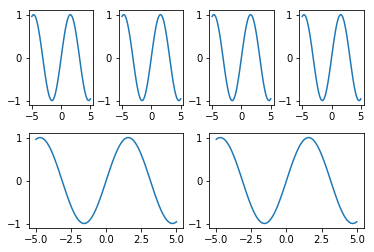#### plt.subplot怎么设置才能每行图的个数不一样？

统计/机器学习 数据可视化 Python    浏览次数：1317        分享

0

plt.subplot(m, n, i)是画出m乘n个子图，每行都是n个图。robin_he   2019-06-18 15:11

##### 1个回答
5

import matplotlib.pyplot as plt
import numpy as np
# 初始化x和y
x = np.linspace(-5, 5, 100)
y = np.sin(x)

# 生成格子，2行4列
grid = plt.GridSpec(2, 4, wspace=0.4, hspace=0.3)
# 第一行的图，每图占一列
plt.subplot(grid[0, 0])
plt.plot(x, y)
plt.subplot(grid[0, 1])
plt.plot(x, y)
plt.subplot(grid[0, 2])
plt.plot(x, y)
plt.subplot(grid[0, 3])
plt.plot(x, y)
# 第二行的图，每图占两列
plt.subplot(grid[1, :2])
plt.plot(x, y)
plt.subplot(grid[1, 2:])
plt.plot(x, y)

plt.show()SofaSofa数据科学社区DS面试题库 DS面经strong.man   2019-07-17 15:11相关主题